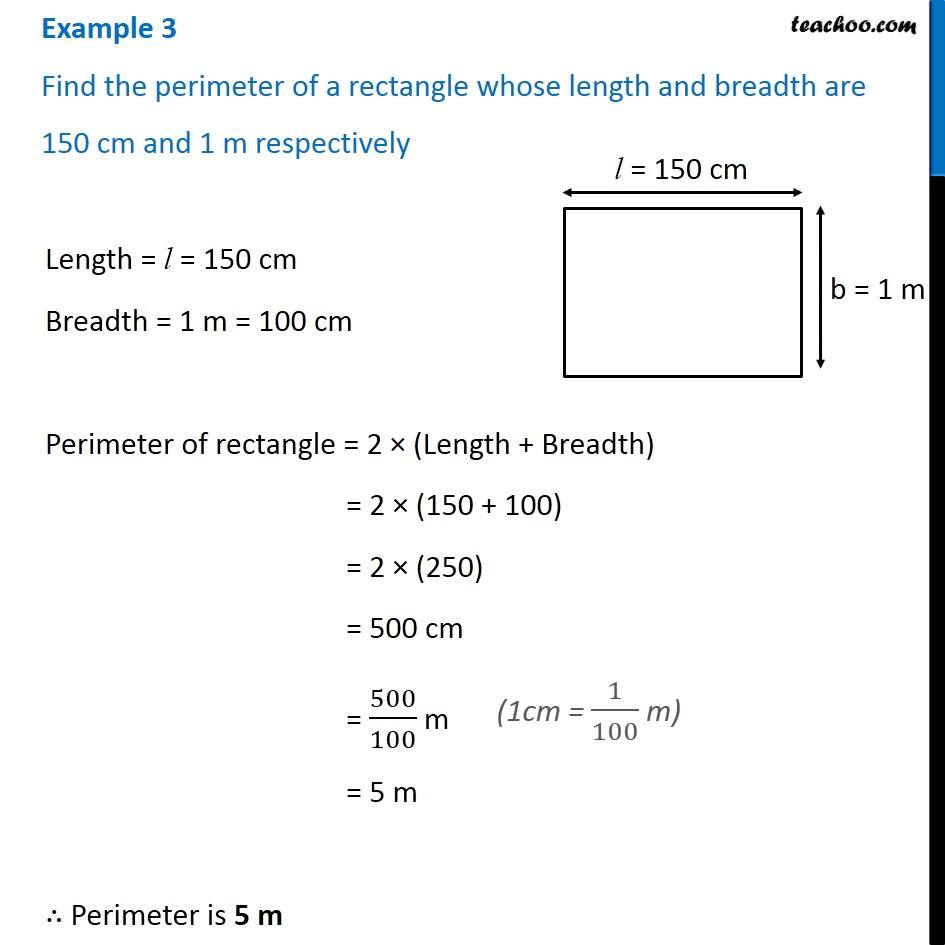Perimeter of Rectangle

Chapter 10 Class 6 Mensuration
Concept wiseGet live Maths 1-on-1 Classs - Class 6 to 12

### Transcript

Example 3 Find the perimeter of a rectangle whose length and breadth are 150 cm and 1 m respectively Length = l = 150 cm Breadth = 1 m = 100 cm Perimeter of rectangle = 2 × (Length + Breadth) = 2 × (150 + 100) = 2 × (250) = 500 cm = 500/100 m = 5 m ∴ Perimeter is 5 m (1cm = 1/100 m)